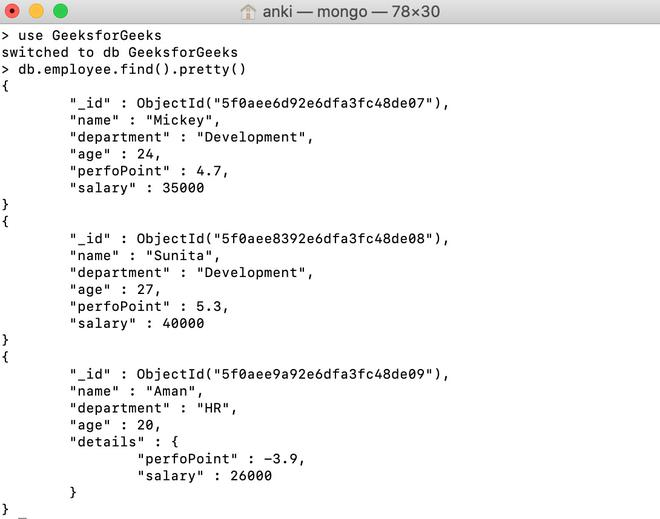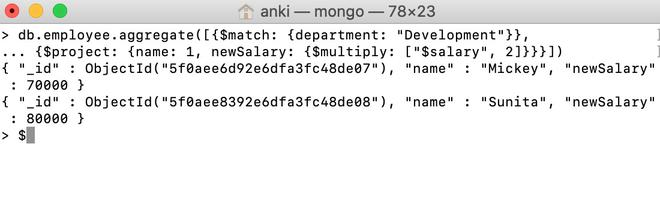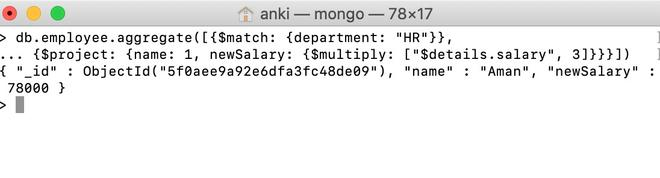GFG App
Open AppBrowser
Continue

MongoDB provides different types of arithmetic expression operators that are used in the aggregation pipeline stages and \$multiply operator is one of them. This operator is used to multiply one number to another number and returns the result.

Syntax:

```{ \$multiply: [ <expression1>, <expression2>, ... <expressionN> ] }
```

Here, in this operator, the arguments passed in an array. The expression must be a valid expression until it resolves to a number.

Examples:

In the following examples, we are working with:

Database: GeeksforGeeks

Collection: employee

Document: three documents that contain the details of the employees in the form of field-value pairs.Multiply using \$multiply operator:

In this example, we are going to multiply the salary of the development department’s employees by 2.

```db.employee.aggregate([{\$match: {department: "Development"}},
... {\$project: {name: 1, newSalary: {\$multiply: ["\$salary", 2]}}}])

```Multiple using \$multiply operator in the embedded document:

In this example, we are going to multiply the salary of the HR department’s employees by 3.

```db.employee.aggregate([{\$match: {department: "HR"}},
... {\$project: {name: 1, newSalary: {\$multiply: ["\$details.salary", 3]}}}])

```My Personal Notes arrow_drop_up